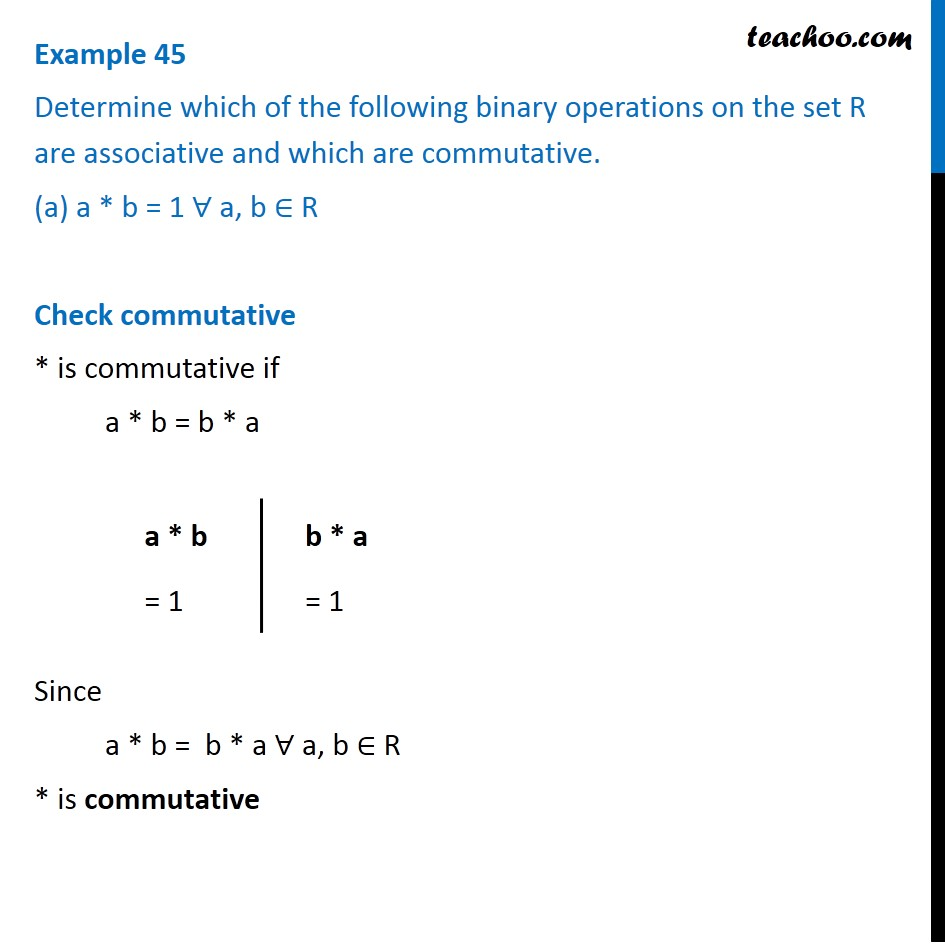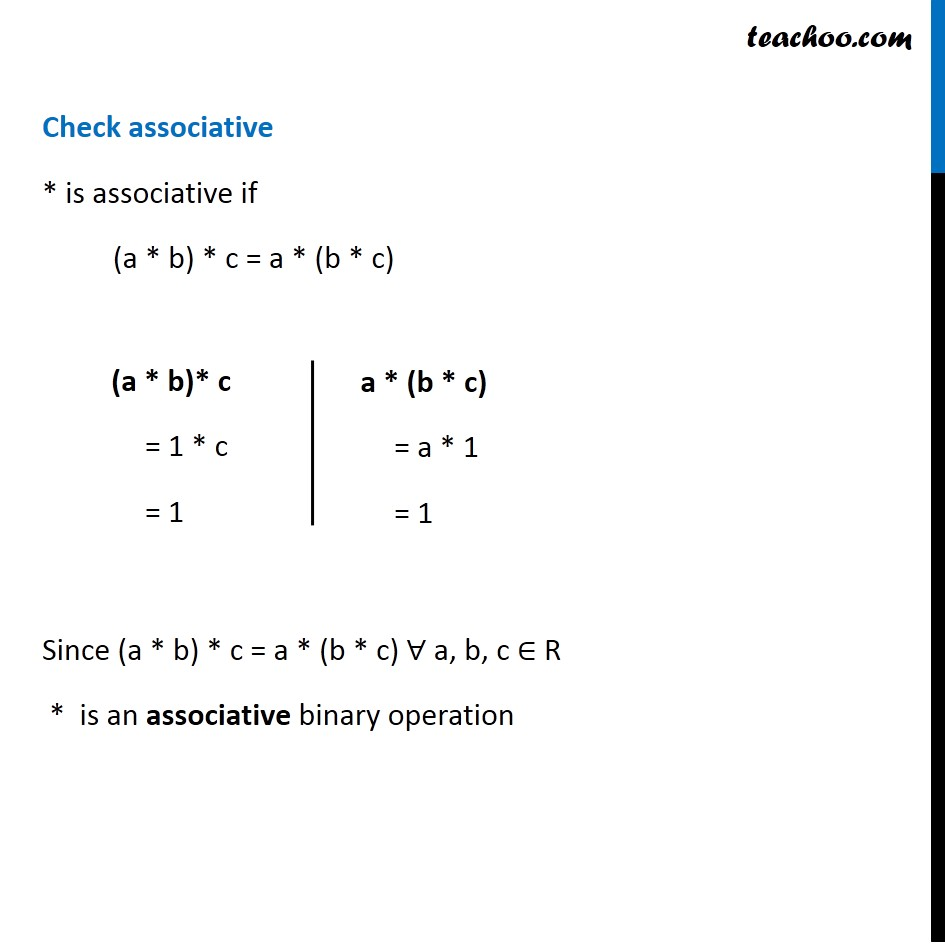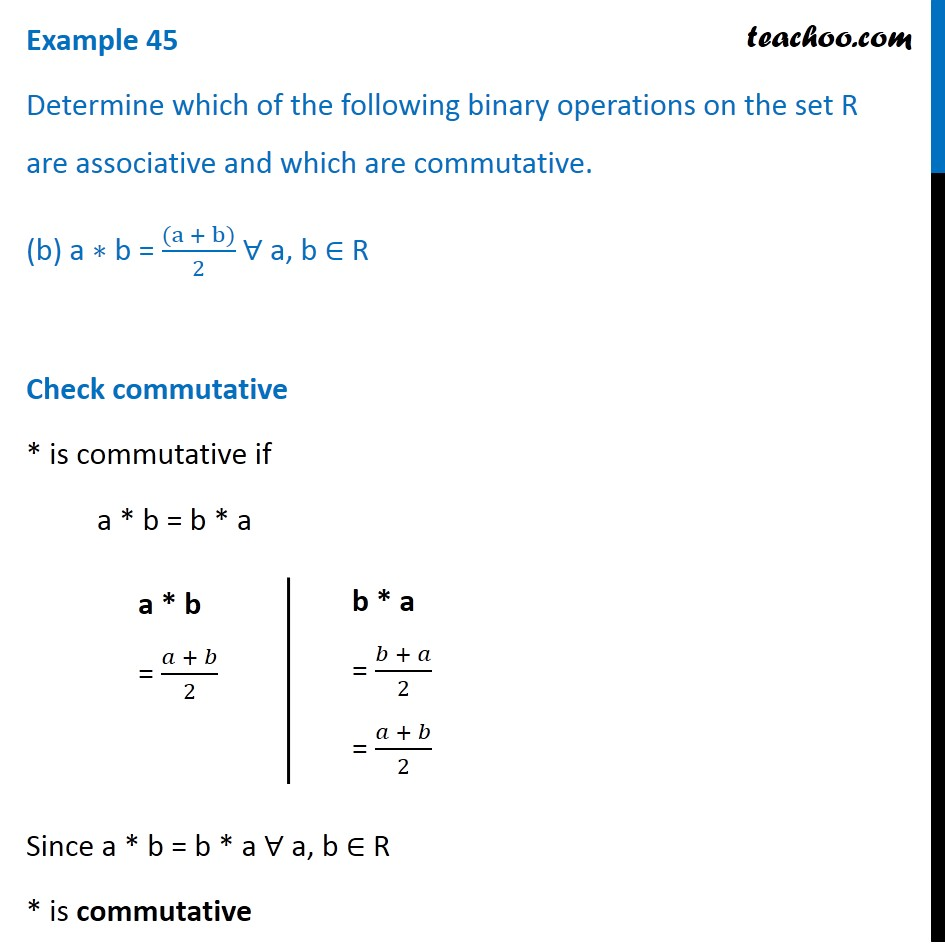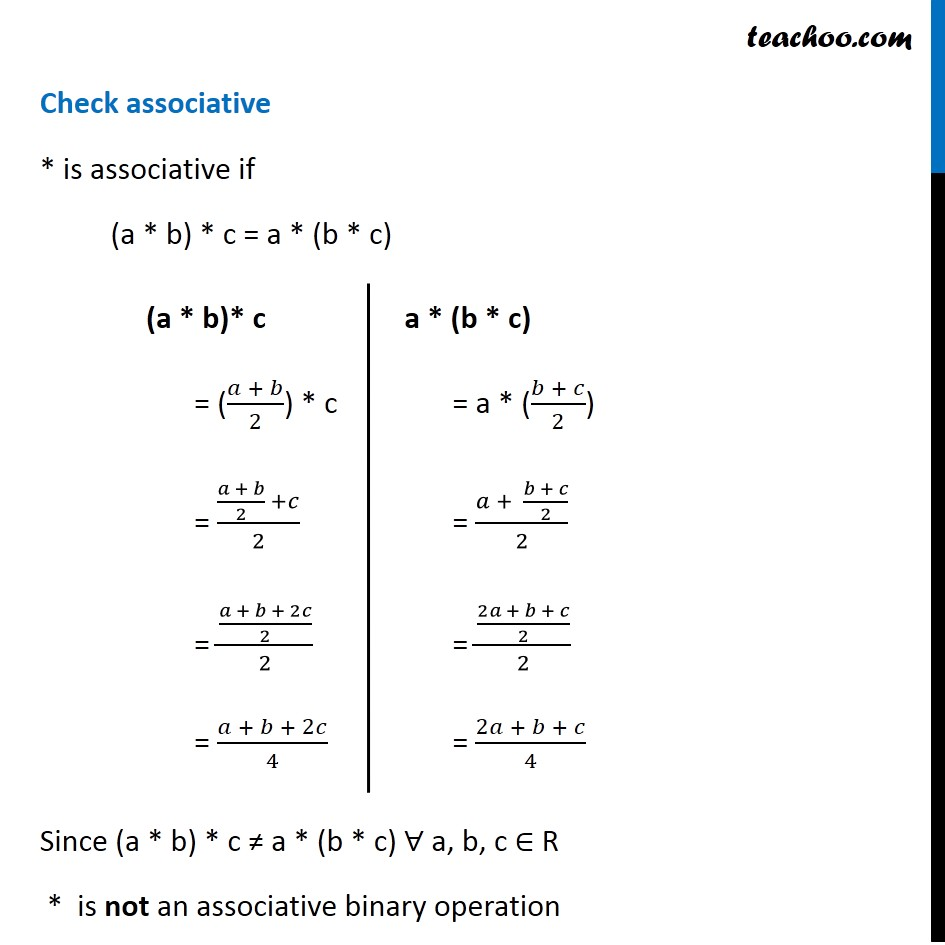Subscribe to our Youtube Channel - https://you.tube/teachoo

1. Chapter 1 Class 12 Relation and Functions
2. Serial order wise
3. Examples

Transcript

Example 45 Determine which of the following binary operations on the set R are associative and which are commutative. (a) a * b = 1 ∀ a, b ∈ R Check commutative * is commutative if a * b = b * a Since a * b = b * a ∀ a, b ∈ R * is commutative a * b = 1 Check associative * is associative if a (a * b) * c = a * (b * c) Since (a * b) * c = a * (b * c) ∀ a, b, c ∈ R * is an associative binary operation Check associative * is associative if a (a * b) * c = a * (b * c) Since (a * b) * c = a * (b * c) ∀ a, b, c ∈ R * is an associative binary operation a * (b * c) = a * 1 = 1 Example 45 Determine which of the following binary operations on the set R are associative and which are commutative. (b) a ∗ b = ((a + b))/2 ∀ a, b ∈ R Check commutative * is commutative if a * b = b * a Since a * b = b * a ∀ a, b ∈ R * is commutative a * b = (𝑎 + 𝑏)/2 b * a = (𝑏 + 𝑎)/2 = (𝑎 + 𝑏)/2 Check associative * is associative if (a * b) * c = a * (b * c) Since (a * b) * c ≠ a * (b * c) ∀ a, b, c ∈ R * is not an associative binary operation (a * b)* c = ((𝑎 + 𝑏)/2) * c = ((𝑎 + 𝑏)/2 +𝑐)/2 = ((𝑎 + 𝑏 + 2𝑐)/2)/2 = (𝑎 + 𝑏 + 2𝑐)/4 a * (b * c) = a * ((𝑏 + 𝑐)/2) = (𝑎 + (𝑏 + 𝑐)/2)/2 = ((2𝑎 + 𝑏 + 𝑐)/2)/2 = (2𝑎 + 𝑏 + 𝑐)/4

Examples